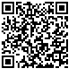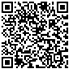## GMAT数学需要积累的重点词汇有哪些？GMAT练习有方

2019-08-12 13:35:37浏览量：530

启德考培小编为大家带来GMAT数学需要积累的重点词汇文章，希望对大家GMAT备考有所帮助。更多精彩尽请关注启德考培官网。

01、词汇积累

angle of greatest slope 最大斜率的角

angle of inclination 倾斜角

angle of intersection 相交角;交角

angle of projection 投射角

(概率)加法定律

angle of rotation 旋转角

02、词汇积累

auxiliary circle 辅助圆

binomial 二项式

auxiliary equation 辅助方程

binomial distribution 二项分佈

average 平均;平均数;平均值

binomial expression 二项式

average speed 平均速率

binomial series 二项级数

axiom 公理

binomial theorem 二项式定理

03、词汇积累

axiom of existence 存在公理

bisect 平分;等分

axiom of extension 延伸公理

bisection method 分半法;分半方法

axiom of inclusion 包含公理

bisector 等分线

Boolean algebra 布尔代数

chain rule 链式法則

boundary condition 边界条件界

chance 机会

04、词汇积累

boundary line 界(线);边界

change of axes 坐标轴的变换

change of coordinates 坐标轴的变换

bounded 有界的

change of base 基的变换

bounded above 有上界的;上有界的

bounded below 有下界的;下有界的

change of subject 主项变换

bounded function 有界函數

change of variable 换元

05、词汇积累

bounded sequence 有界序列

characteristic equation 特征方程

brace 大括号

characteristic function 特征函数

bracket 括号

broken line graph 折线圆

characteristic of logarithm 对数的首数

characteristic root 特征

chart 圆，圆表

以上就为大家整理的GMAT数学需要积累的重点词汇，更多精彩内容，请关注启德考培官网。

## 猜你喜欢## 留学费用查询## 分数兑换器## 雅思/托福测试## 星座对应留学国家

12星座分别适合去哪个国家留学呢，赶快来测测吧